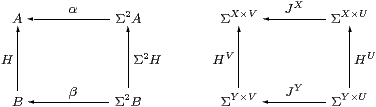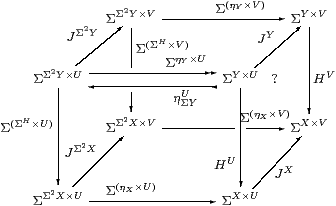## 3  Algebras and homomorphisms

Notation 3.1 The adjunction in Proposition 2.11 gives rise to a strong monad with

1. multiplication µXηΣX4 X→Σ2 X given by F↦λ φ.FF.Fφ), and
2. strength σΓ,X:Γ×Σ2 X→Σ2(Γ× X) given by γ,F↦λψ.Fx.ψ(γ,x)),

satisfying six equations. Eugenio Moggi [Mog91] demonstrated how strong monads can be seen as notions of computation, giving a (“let ”) calculus in which µ is used to interpret composition and σ to substitute for parameters. Constructions similar to ours can be performed in this generality.

However, rather than develop an abstract theory of monads, our purpose is to demonstrate the relevance of one particular monad and show how it accounts for the intuitions in Section 1. The associativity law for µ involves Σ6 X, but we certainly don’t want to compute with such λ-terms unless it is absolutely necessary! In fact we can largely avoid using σ and µ in this work.

Definition 3.2 An Eilenberg–Moore algebra for the monad is an object A of C together with a morphism α:Σ2 AA such that ηA;α=idA and µA;α=Σ2 α;α.Lemma 3.3 For any object X, (ΣXηX) is an algebra.

Proof    The equations are just those in Lemma 2.10, although the one involving µ is Σ(−) applied to the naturality equation for η with respect to ηX.         ▫

These are the only algebras that will be used in this paper, but [B] shows how general algebras may be regarded as the topologies on subspaces that are defined by an axiom of comprehension. In other words, all algebras are of this form, but with a generalised definition of the type X.

Definition 3.4 We shall show that the following are equivalent (when AX etc.):

1. For any two algebras (A,α) and (B,β), a C-morphism H:BA is called an (Eilenberg–Moore) homomorphism if β;H2 H;α, as in the square on the left.2. A morphism HY→ΣX is called central if for every morphism JU→ΣV, the equation JY;HV=HU;JX holds, as in the square on the right.

We must be careful with the notation JX, as it is ambiguous which way round the product is in the exponent.

Proposition 3.5 If H is a homomorphism or central, and invertible in C, then its inverse is also a homomorphism or central, respectively.         ▫

Lemma 3.6 For any map f:XY in C, the map ΣfY→ΣX is both a homomorphism and central.

Proof    It is a homomorphism by naturality of η, and central by naturality of J(−), with respect to f.         ▫

Lemma 3.7 Every homomorphism HY→ΣX is central.Proof    The front and back faces commute since H is a homomorphism. The left, bottom and top faces commute by naturality of J(−) with respect to ΣH, ηX and ηY. Using the split epi, the right face commutes, but this expresses centrality.         ▫

Remark 3.8 To obtain the converse, we ought first to understand how monads provide a “higher order” account of infinitary algebraic theories [Lin69]. The infinitary theory corresponding to our monad has an operation-symbol J of arity U for each morphism JU→Σ (for example, the additional lattice structure consists of morphisms ∧:Σ2→Σ and ⋁:Σ→Σ). Then the centrality square is the familiar rule for a homomorphism H to commute with the symbol J.

More generally, H commutes with JU→ΣV iff it is a homomorphism parametrically with respect to a V-indexed family of U-ary operation-symbols. (So, for example, a map J3→Σ2 denotes a pair of ternary operations.) The next two results are examples of this idea, and we apply it to the lattice structure in the topological interpretation in Proposition 5.5.

Lemma 3.9 HY→ΣX is a homomorphism with respect to all constants σ∈Σ,

i.e.   σ:Σ⊢ Hy.σ) = λ x.σ,   iff  Σ!Y;H!X.Proof    J=∼id:1→ΣΣ denotes a Σ-indexed family of constants, for which the centrality square is as shown.         ▫

The following proof is based on the idea that each rT B corresponds to an operation-symbol of arity B that acts on A as rA:ABA by f↦ α(T f r). The rectangle says that K is a homomorphism for this operation-symbol, but it does so for all rT B simultaneously by using the exponential (−)T B.

Thielecke [Thi97a, Lemma 5.2.5] proves this result using his CPS λ-calculus, whilst Selinger [Sel01, Lemma 2.10] gives another categorical proof. They do so with surprisingly little comment, given that it is the Completeness Theorem corresponding to the easy Soundness Lemma 3.7.

Theorem 3.10 All central maps are homomorphisms.

Proof    Let H:BYAX. Writing T for both the functor ΣΣ(−) and its effect on internal hom-sets, consider the rectanglein which the left-hand square says that T preserves composition and the right-hand square that H is an Eilenberg–Moore homomorphism. To deduce the latter, it suffices to show that the rectangle commutes at idBB (which T takes to idTBTB).

Re-expanding, the map along the bottom is ΣX× B→ΣΣ2 X×Σ2 B→ ΣX×Σ2 B by

 θ↦λ F G.G(λ b.F(θ b)) ↦λ x G.G(λ b.ηX x (θ b)) =λ x G.G(λ b.θ b x),

which is ηΣBX, and similarly the top map is ηΣBY. Thus the rectangle says that HY→ΣX is central with respect to ηΣB, which was the hypothesis.         ▫

Notation 3.11 We write Alg (or sometimes AlgC) for the category of Eilenberg–Moore algebras and homomorphisms.

Lemma 3.12 For any object X, (ΣΣXX) is the free algebra on X. In particular, (Σ, Ση1) is the initial algebra.         ▫

Definition 3.13 The full subcategory of Alg consisting of free algebras is known as the Kleisli category for the monad.

As ΣΣX is free on X, the name of this object in the traditional presentation of the Kleisli category is abbreviated to X, and the homomorphism XK Y (i.e. ΣΣX→ΣΣY) is named by the ordinary map f:X→ΣΣY. This presentation is complicated by the fact that the identity on X is named by ηX and the composite of f:XK Y and g:YK Z by f2 gZ.

Using the double exponential transpose (Proposition 2.11), this homomorphism is more simply written as an arbitrary map FY→ΣX, with the usual identity and composition.

The (opposites of the) categories composed of morphisms FY→ΣX, in the cases where F is an arbitrary C-map (as for the Kleisli category), or required to be a homomorphism, will be developed in Section 6.